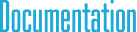#SpatialVector6< TYPE > Class Template Reference

## Detailed Description

### template<typename TYPE> class cy::SpatialVector6< TYPE >

6D spatial vector (for 3D).

This class is both for spatial motion vectors and spatial force vectors.

`#include <cySpatial.h>`

## Public Member Functions

Constructors
SpatialVector6 ()

SpatialVector6 (const Point3< TYPE > &p1, const Point3< TYPE > &p2)

SpatialVector6 (TYPE a1, TYPE a2, TYPE a3, TYPE b1, TYPE b2, TYPE b3)

SpatialVector6 (const SpatialVector6 &v)

Initialization methods
void Set (const Point3< TYPE > &p1, const Point3< TYPE > &p2)

void Set (TYPE a1, TYPE a2, TYPE a3, TYPE b1, TYPE b2, TYPE b3)

void Zero ()

Transpose methods
void SetTranspose ()

SpatialVector6 Transpose () const

Unary operators
SpatialVector6 operator- () const

Binary operators
SpatialVector6 operator+ (const SpatialVector6 &s) const

SpatialVector6 operator- (const SpatialVector6 &s) const

SpatialVector6 operator* (TYPE t) const

TYPE operator* (const SpatialVector6 &s) const

Assignment operators
void operator= (const SpatialVector6 &v)

void operator+= (const SpatialVector6 &s)

void operator-= (const SpatialVector6 &s)

void operator*= (TYPE t)

Components
Point3< TYPE > a

Point3< TYPE > b

## § operator+()

 SpatialVector6 operator+ ( const SpatialVector6< TYPE > & s ) const

Scalar product of two vectors. Note that one of the vectors should be motion vector ant the other should be a force vector. Otherwise, scalar product is not defined in spatial vector algebra.

## § operator-()

 SpatialVector6 operator- ( const SpatialVector6< TYPE > & s ) const

Scalar product of two vectors. Note that one of the vectors should be motion vector ant the other should be a force vector. Otherwise, scalar product is not defined in spatial vector algebra.

## § operator*() [1/2]

 SpatialVector6 operator* ( TYPE t ) const

Scalar product of two vectors. Note that one of the vectors should be motion vector ant the other should be a force vector. Otherwise, scalar product is not defined in spatial vector algebra.

## § operator*() [2/2]

 TYPE operator* ( const SpatialVector6< TYPE > & s ) const

Scalar product of two vectors. Note that one of the vectors should be motion vector ant the other should be a force vector. Otherwise, scalar product is not defined in spatial vector algebra.